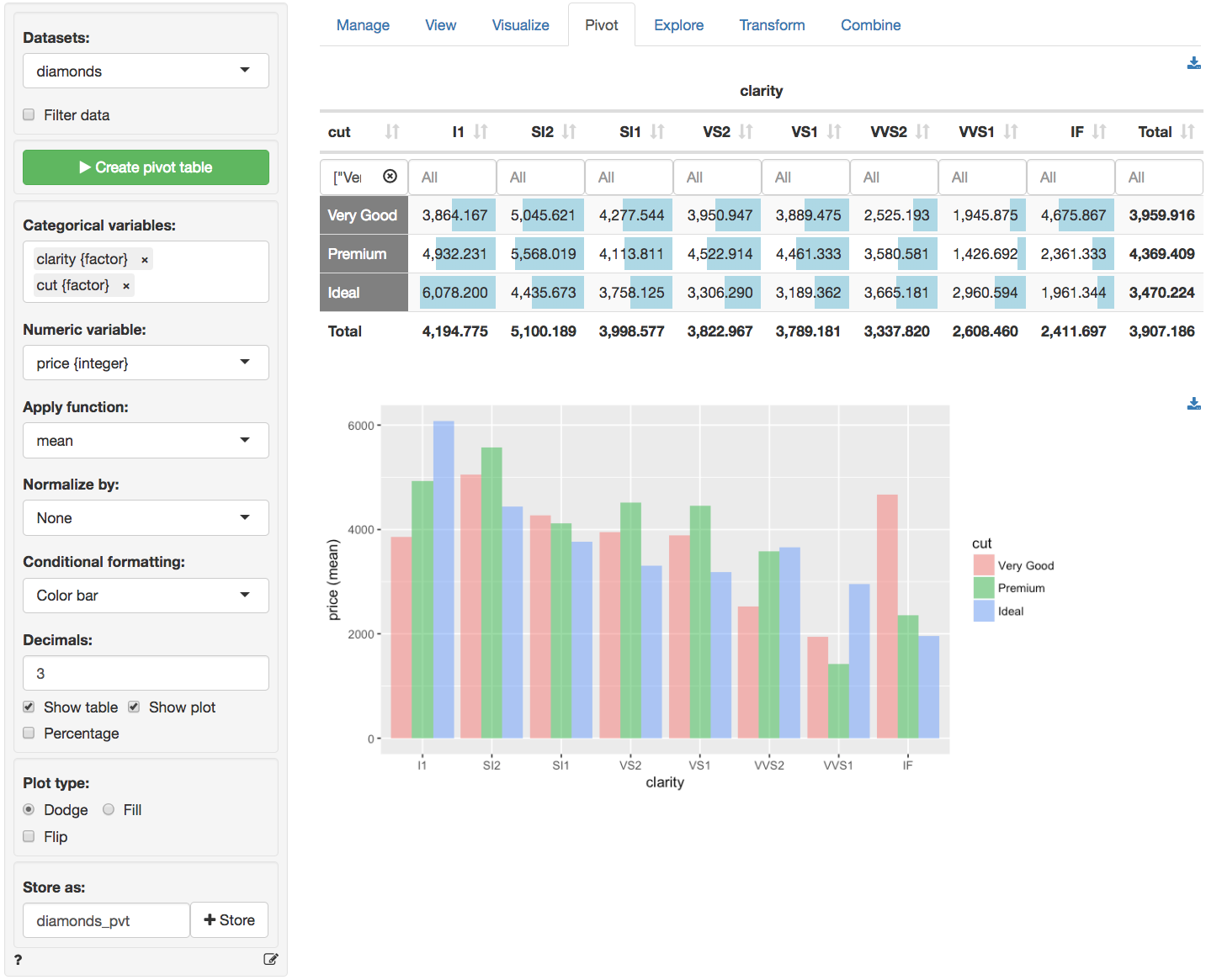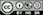Create pivot tables to explore your data

If you have used pivot-tables in Excel the functionality provided in the Data > Pivot tab should be familiar to you. Similar to the Data > Explore tab, you can generate summary statistics for variables in your data. You can also generate frequency tables. Perhaps the most powerful feature in Data > Pivot is that you can easily describe the data by one or more other variables.

For example, with the diamonds data loaded, select clarity and cut from the Categorical variables drop-down. The categories for the first variable will be the column headers but you can drag-and-drop the selected variables to change their ordering. After selecting these two variables, and clicking on the Create pivot table button, a frequency table of diamonds with different levels of clarity and quality of cut is shown. Choose Row, Column, or Total from the Normalize by drop-down to normalize cell frequencies or create an index from a summary statistic by the row, column, or overall total. If a normalize option is selected it can be convenient to check the Percentage box to express the numbers as percentages. Choose Color bar or Heat map from the Conditional formatting drop-down to emphasize the highest frequency counts.

It is also possible to summarize numerical variables. Select price from the Numeric variables drop-down. This will create the table shown below. Just as in the Data > View tab you can sort the table by clicking on the column headers. You can also use sliders (e.g., click in the input box below I1) to limit the view to values in a specified range. To view only information for diamonds with a Very good, Premium or Ideal cut click in the input box below the cut header.Below you will find a brief description of several functions available from the Apply function dropdown menu. Most functions, however, will be self-explanatory.

• n calculates the number of observations, or rows, in the data or in a group if a Group by variable has been selected (n uses the length function in R)
• n_distinct calculates the number of distinct values
• n_missing calculates the number of missing values
• cv is the coefficient of variation (i.e., mean(x) / sd(x))
• sd and var calculate the sample standard deviation and variance for numeric data
• me calculates the margin of error for a numeric variable using a 95% confidence level
• prop calculates a proportion. For a variable with only values 0 or 1 this is equivalent to mean. For other numeric variables it captures the occurrence of the maximum value. For a factor it captures the occurrence of the first level.
• sdprop and varprop calculate the sample standard deviation and variance for a proportion
• meprop calculates the margin of error for a proportion using a 95% confidence level
• sdpop and varpop calculate the population standard deviation and variance

You can also create a bar chart based on the generated table (see image above). To download the table in csv format or the plot in png format click the appropriate download icon on the right.

Note that when a categorical variable (factor) is selected from the Numeric variable(s) dropdown menu it will be converted to a numeric variable if required for the selected function(s). If the factor levels are numeric these will be used in all calculations. Since the mean, standard deviation, etc. are not relevant for non-binary categorical variables, these will be converted to 0-1 (binary) variables where the first level is coded as 1 and all other levels as 0.

### Filter data

Use the Filter data box to select (or omit) specific sets of rows from the data to tabulate. See the help file for Data > View for details.

### Store

The created pivot table can be stored in Radiant by clicking the Store button. This can be useful if you want do additional analysis on the table or to create plots of the summarized data in Data > Visualize. To download the table to csv format click the download icon on the top-right.

### Report > Rmd

Add code to Report > Rmd to (re)create the pivot table by clicking the icon on the bottom left of your screen or by pressing ALT-enter on your keyboard.

If a plot was created it can be customized using ggplot2 commands (e.g., plot(result) + labs(title = "Pivot graph")). See Data > Visualize for details.

### R-functions

For an overview of related R-functions used by Radiant to create pivot tables see Data > Pivot

© Vincent Nijs (2019)